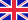•Paths and Cycles in Digraphs

This book is intended to investigate a famous conjecture in Graph Theory proposed by Caccetta and Haggkvist in 1978. The book demonstrates the conjecture which has two forms, and the equivalence of the two forms of the conjecture is proven. The conjecture... Viac o knihe

Na objednávku, dodanie 2-4 týždne

42.84 €

bežná cena: 50.40 €

O knihe

This book is intended to investigate a famous conjecture in Graph Theory proposed by Caccetta and Haggkvist in 1978. The book demonstrates the conjecture which has two forms, and the equivalence of the two forms of the conjecture is proven. The conjecture relates the outdegree of the vertices in a digraph along with the existence of a short directed cycle of certain length in that digraph. Here, two main approaches to resolve the conjecture will be described. The first approach is by Hamidoune to prove the conjecture if the outdegree of each vertex in the digraph is at most three. The second approach is by Hoang and Reed to prove the conjecture if the outdegree of each vertex in the digraph is at most five. Both these approaches are investigated in detail and new techniques are developed in order to be used for subsequent research. Occasionally, the techniques used to prove the conjecture if the outdegree of each vertex is five can also be used to prove the conjecture if the outdegree of each vertex is six.

• Vydavateľstvo: LAP LAMBERT Academic Publishing
• Rok vydania: 2012
• Formát: Paperback
• Rozmer: 220 x 150 mm
• Jazyk: Anglický jazyk
• ISBN: 9783659184284

Generuje redakčný systém BUXUS CMS spoločnosti ui42.